# Exponential & Logarithmic Functions in Trigonometry: Tutoring Solution Chapter Exam

Exam Instructions:

Choose your answers to the questions and click 'Next' to see the next set of questions. You can skip questions if you would like and come back to them later with the yellow "Go To First Skipped Question" button. When you have completed the practice exam, a green submit button will appear. Click it to see your results. Good luck!

### Page 2

#### Question 6 6. Solve the exponential equation below for x: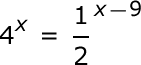#### Question 10 10. Evaluate the logarithm below: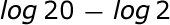### Page 3

#### Question 11 11. Condense the logarithm below: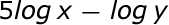#### Question 14 14. What kind of shift happens with this function?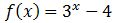#### Question 15 15. Solve the exponential equation below for x: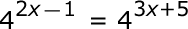### Page 4

#### Question 17 17. Evaluate.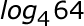#### Question 18 18. Evaluate.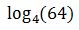#### Question 19 19. Rewrite the following in its inverse form.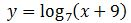### Page 5

#### Question 22 22. What transformation happens with this function?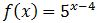#### Question 24 24. Which logarithmic property is shown below?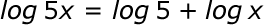#### Question 25 25. Solve, to 2 decimal places, the exponential equation below for x: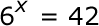### Page 6

#### Question 26 26. Simplify the following expression.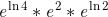#### Question 27 27. Evaluate to 2 decimal places.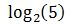#### Question 28 28. Solve the differential equation for your bank balance given as follows.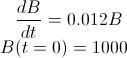#### Question 30 30. The following differential equation represents the change in population as a function of time. What does the 0.03 represent in this equation?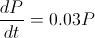#### Exponential & Logarithmic Functions in Trigonometry: Tutoring Solution Chapter Exam Instructions

Choose your answers to the questions and click 'Next' to see the next set of questions. You can skip questions if you would like and come back to them later with the yellow "Go To First Skipped Question" button. When you have completed the practice exam, a green submit button will appear. Click it to see your results. Good luck!

Support